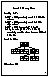# 1. M-way Search TreesA binary search tree has one value in each node and two subtrees. This notion easily generalizes to an M-way search tree, which has (M-1) values per node and M subtrees. M is called the degree of the tree. A binary search tree, therefore, has degree 2.

In fact, it is not necessary for every node to contain exactly (M-1) values and have exactly M subtrees. In an M-way subtree a node can have anywhere from 1 to (M-1) values, and the number of (non-empty) subtrees can range from 0 (for a leaf) to 1+(the number of values). M is thus a fixed upper limit on how much data can be stored in a node.

The values in a node are stored in ascending order, V1 < V2 < ... Vk (k <= M-1) and the subtrees are placed between adjacent values, with one additional subtree at each end. We can thus associate with each value a `left' and `right' subtree, with the right subtree of Vi being the same as the left subtree of V(i+1). All the values in V1's left subtree are less than V1 ; all the values in Vk's subtree are greater than Vk; and all the values in the subtree between V(i) and V(i+1) are greater than V(i) and less than V(i+1).

For example, here is a 3-way search tree:

``
In our examples it will be convenient to illustrate M-way trees using a small value of M. But bear in mind that, in practice, M is usually very large. Each node corresponds to a physical block on disk, and M represents the maximum number of data items that can be stored in a single block. M is maximized in order to speedup processing: to move from one node to another involves reading a block from disk - a very slow operation compared to moving around a data structure stored in memory.The algorithm for searching for a value in an M-way search tree is the obvious generalization of the algorithm for searching in a binary search tree. If we are searching for value X are and currently at node consisting of values V1...Vk, there are four possible cases that can arise:

1. If X < V1, recursively search for X in V1's left subtree.
2. If X > Vk, recursively search for X in Vk's right subtree.
3. If X=Vi, for some i, then we are done (X has been found).
4. the only remaining possibility is that, for some i, Vi < X < V(i+1). In this case recursively search for X in the subtree that is in between Vi and V(i+1).
For example, suppose we were searching for 68 in the tree above. At the root, case (2) would apply, so we would continue the search in V2's right subtree. At the root of this subtree, case (4) applies, 68 is between V1=55 and V2=70, so we would continue to search in the subtree between them. Now case (3) applies, 68=V2, so we are done. If we had been searching for 69, exactly the same processing would have occurred down to the last node. At that point, case (2) would apply, but the subtree we want to search in is empty. Therefore we conclude that 69 is not in the tree.

Other the algorithms for binary search trees - insertion and deletion - generalize in a similar way. As with binary search trees, inserting values in ascending order will result in a degenerate M-way search tree; i.e. a tree whose height is O(N) instead of O(logN). This is a problem because all the important operations are O(height), and it is our aim to make them O(logN). One solution to this problem is to force the tree to be height-balanced; we sketched this last lecture for binary search trees and now we will examine it in detail for M-way search trees.